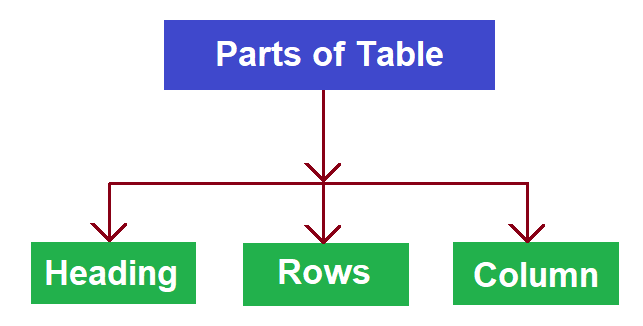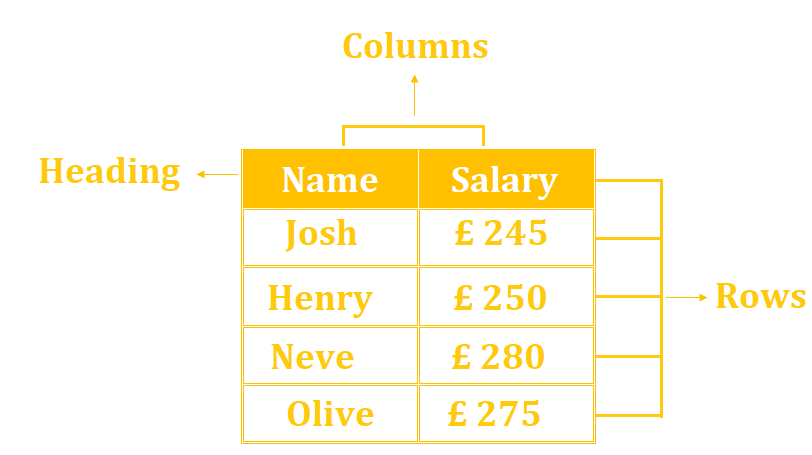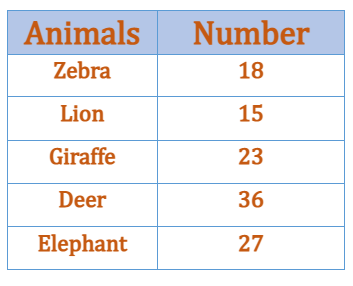# Math > Year 3

## Interpreting and Presenting data in tables

What is a Table?
A table is a type of graph that presents data in tabular form. Tables are made up of rows and columns.

A table has 3 parts.Example: Here is a table showing the weekly salary of 4 different persons.The top row shows the heading i.e., the details of the data being represented in the table.

Rows are horizontal cells.
Columns are the vertical cells.

Let’s try a question.

The given table shows the number of different animals in a Zoo.How many more elephants are there in the zoo as compared to Giraffes?

The number of Elephants = 27

Number of Giraffes = 23

To find out the difference we subtract i.e., 27 – 23 = 4

There are 4 more elephants.

//